## Cubic Spline Interpolation

### Cubic Spline Interpolant of Smooth Data

Suppose you want to interpolate some smooth data, e.g., to

```rng(6), x = (4*pi)*[0 1 rand(1,15)]; y = sin(x); ```

You can use the cubic spline interpolant obtained by

```cs = csapi(x,y); ```

and plot the spline, along with the data, with the following code:

```fnplt(cs); hold on plot(x,y,'o') legend('cubic spline','data') hold off ```

This produces a figure like the following.

Cubic Spline Interpolant of Smooth DataThis is, more precisely, the cubic spline interpolant with the not-a-knot end conditions, meaning that it is the unique piecewise cubic polynomial with two continuous derivatives with breaks at all interior data sites except for the leftmost and the rightmost one. It is the same interpolant as produced by the MATLAB® `spline` command, `spline(x,y)`.

### Periodic Data

The sine function is 2π-periodic. To check how well your interpolant does on that score, compute, e.g., the difference in the value of its first derivative at the two endpoints,

```diff(fnval(fnder(cs),[0 4*pi])) ans = -.0100 ```

which is not so good. If you prefer to get an interpolant whose first and second derivatives at the two endpoints, `0` and `4*pi`, match, use instead the command `csape` which permits specification of many different kinds of end conditions, including periodic end conditions. So, use instead

```pcs = csape(x,y,'periodic'); ```

for which you get

```diff(fnval(fnder(pcs),[0 4*pi])) ```

Output is `ans = 0` as the difference of end slopes. Even the difference in end second derivatives is small:

```diff(fnval(fnder(pcs,2),[0 4*pi])) ```

Output is `ans = -4.6074e-015`.

### Other End Conditions

Other end conditions can be handled as well. For example,

```cs = csape(x,[3,y,-4],[1 2]); ```

provides the cubic spline interpolant with breaks at the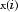and with its slope at the leftmost data site equal to 3, and its second derivative at the rightmost data site equal to -4.

### General Spline Interpolation

If you want to interpolate at sites other than the breaks and/or by splines other than cubic splines with simple knots, then you use the `spapi` command. In its simplest form, you would say `sp = spapi(k,x,y)`; in which the first argument, `k`, specifies the order of the interpolating spline; this is the number of coefficients in each polynomial piece, i.e., 1 more than the nominal degree of its polynomial pieces. For example, the next figure shows a linear, a quadratic, and a quartic spline interpolant to your data, as obtained by the statements

```sp2 = spapi(2,x,y); fnplt(sp2,2), hold on sp3 = spapi(3,x,y); fnplt(sp3,2,'k--'), sp5 = spapi(5,x,y); fnplt(sp5,2,'r-.'), plot(x,y,'o') legend('linear','quadratic','quartic','data'), hold off ```

Spline Interpolants of Various Orders of Smooth Data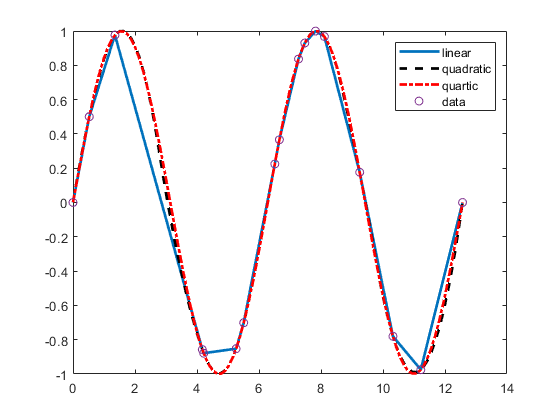Even the cubic spline interpolant obtained from `spapi` is different from the one provided by `csapi` and `spline`. To emphasize their difference, compute and plot their second derivatives, as follows:

```fnplt(fnder(spapi(4,x,y),2)), hold on, fnplt(fnder(csapi(x,y),2),2,'k--'),plot(x,zeros(size(x)),'o') legend('from spapi','from csapi','data sites'), hold off ```

This gives the following graph:

Second Derivative of Two Cubic Spline Interpolants of the Same Smooth Data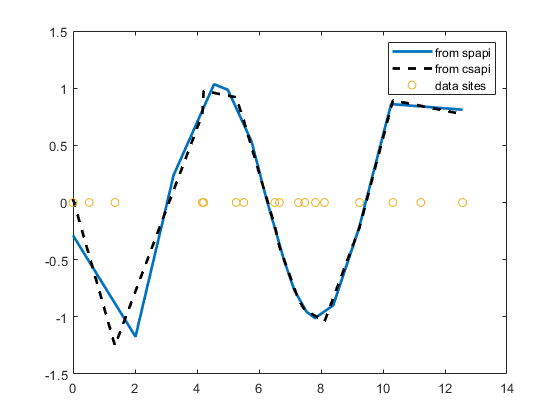Since the second derivative of a cubic spline is a broken line, with vertices at the breaks of the spline, you can see clearly that `csapi` places breaks at the data sites, while `spapi` does not.

### Knot Choices

It is, in fact, possible to specify explicitly just where the spline interpolant should have its breaks, using the command ```sp = spapi(knots,x,y)```; in which the sequence `knots` supplies, in a certain way, the breaks to be used. For example, recalling that you had chosen `y` to be `sin(x)`, the command

```ch = spapi(augknt(x,4,2),[x x],[y cos(x)]); ```

provides a cubic Hermite interpolant to the sine function, namely the piecewise cubic function, with breaks at all the `x(i)`'s, that matches the sine function in value and slope at all the `x(i)`'s. This makes the interpolant continuous with continuous first derivative but, in general, it has jumps across the breaks in its second derivative. Just how does this command know which part of the data value array `[y cos(x)]` supplies the values and which the slopes? Notice that the data site array here is given as `[x x]`, i.e., each data site appears twice. Also notice that `y(i)` is associated with the first occurrence of `x(i)`, and `cos(x(i))` is associated with the second occurrence of `x(i)`. The data value associated with the first appearance of a data site is taken to be a function value; the data value associated with the second appearance is taken to be a slope. If there were a third appearance of that data site, the corresponding data value would be taken as the second derivative value to be matched at that site. See Constructing and Working with B-form Splines for a discussion of the command `augknt` used here to generate the appropriate "knot sequence".

### Smoothing

What if the data are noisy? For example, suppose that the given values are

```noisy = y + .3*(rand(size(x))-.5); ```

Then you might prefer to approximate instead. For example, you might try the cubic smoothing spline, obtained by the command

```scs = csaps(x,noisy); ```

and plotted by

```fnplt(scs,2), hold on, plot(x,noisy,'o'), legend('smoothing spline','noisy data'), hold off ```

This produces a figure like this:

Cubic Smoothing Spline of Noisy Data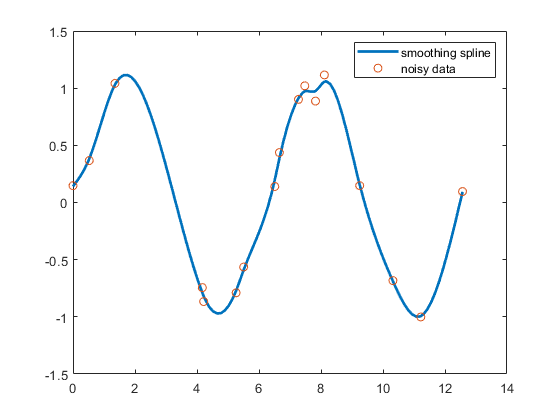If you don't like the level of smoothing done by `csaps(x,y)`, you can change it by specifying the smoothing parameter, `p`, as an optional third argument. Choose this number anywhere between 0 and 1. As `p` changes from 0 to 1, the smoothing spline changes, correspondingly, from one extreme, the least squares straight-line approximation to the data, to the other extreme, the "natural" cubic spline interpolant to the data. Since `csaps` returns the smoothing parameter actually used as an optional second output, you could now experiment, as follows:

```[scs,p] = csaps(x,noisy); fnplt(scs,2), hold on fnplt(csaps(x,noisy,p/2),2,'k--'), fnplt(csaps(x,noisy,(1+p)/2),2,'r:'), plot(x,noisy,'o') legend('smoothing spline','more smoothed','less smoothed',... 'noisy data'), hold off ```

This produces the following picture.

Noisy Data More or Less Smoothed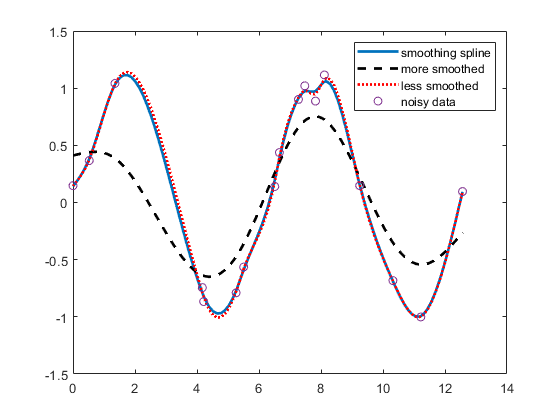At times, you might prefer simply to get the smoothest cubic spline `sp` that is within a specified tolerance `tol` of the given data in the sense that ```norm(noisy - fnval(sp,x))^2 <= tol```. You create this spline with the command ```sp = spaps(x,noisy,tol)``` for your defined tolerance `tol`.

### Least Squares

If you prefer a least squares approximant, you can obtain it by the statement `sp = spap2(knots,k,x,y)`; in which both the knot sequence `knots` and the order `k` of the spline must be provided.

The popular choice for the order is 4, and that gives you a cubic spline. If you have no clear idea of how to choose the knots, simply specify the number of polynomial pieces you want used. For example,

```sp = spap2(3,4,x,y); ```

gives a cubic spline consisting of three polynomial pieces. If the resulting error is uneven, you might try for a better knot distribution by using `newknt` as follows:

```sp = spap2(newknt(sp),4,x,y); ```
﻿